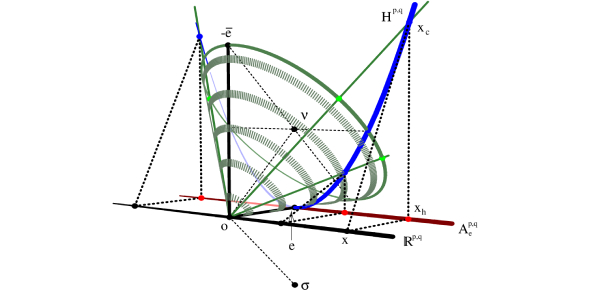# SAT Mathematics: Algebra And Geometry Quiz!

23 Questions | Attempts: 4865
ShareSettingsThis quiz comprises of is a 23-questions that focuses strictly on algebra questions on the SAT Mathematics test. Specifically, this quiz tests your ability to solve simple linear and quadratic algebraic equations. Read the questions carefully and answer. Let's take the quiz.

• 1.
Tom is four years older than Kate. In two years, Kate will be twice as old as Marianne, who is four. How old is Tom?
• A.

10

• B.

12

• C.

14

• D.

16

• E.

18

• 2.
If 2x = 3(x-2), 6x + 3 = ?
• A.

0

• B.

6

• C.

15

• D.

39

• E.

42

• 3.
Which of the following is not a rational number?
• A.

The square root of 4

• B.

-7

• C.

3.14

• D.

The square root of 2

• E.

144

• 4.
If 2(x-3) = 14, what is x^2 - 6x + 9?
• A.

7

• B.

14

• C.

49

• D.

108

• E.

121

• 5.
Which of the following values of x will yield an integer value for 1 ----- x^2-3
• A.

-3

• B.

Square root of 3/4

• C.

Square root of 7/2

• D.

4/3

• E.

7/2

• 6.
Which of the following could not the product of an even number and an odd number?
• A.

42

• B.

22

• C.

15

• D.

18

• E.

1004

• 7.
Given that there are X red marbles, X-4 blue marbles, and 3X+2 green marbles in a bag of exactly 18 combined red, blue, and green marbles, what is the chance of randomly selecting a red or blue marble?
• A.

1/9

• B.

2/9

• C.

1/3

• D.

2/3

• E.

5/9

• 8.
Thomas has twice the amount of money that John does. Terry has 40 dollars less than Thomas. If the amount of money that John has is "J," how much money would Terry have in terms of J if she received a 20-dollar gift?
• A.

2*J+20

• B.

2-J

• C.

20*J-2

• D.

2*J-20

• E.

2/J + 20

• 9.
If x^3 = 12, x^6 =
• A.

18

• B.

24

• C.

96

• D.

98

• E.

144

• 10.
A - (4 - a) = 3a + 3. a = ?
• A.

-7

• B.

-3

• C.

0

• D.

3

• E.

7

• 11.
Two positive integers have a product of 96. The difference between the largest and the smallest of them is 20. Which of the following is the sum of the integers?
• A.

14

• B.

20

• C.

24

• D.

28

• E.

36

• 12.
At how many points do the lines given by the equations 2x + 2y = 3 and y = -x + 1 intersect?
• A.

0

• B.

1

• C.

2

• D.

3

• E.

Infinitely many

• 13.
John wishes to build a square fence with an area of 121 square yards. What is the perimeter of the fence, in yards?
• A.

11

• B.

12

• C.

33

• D.

44

• E.

484

• 14.
If F(x) = 2x + 2 and G(x) = x^2 - 1, what is F(G(3)) - G(F(3))?
• A.

-47

• B.

-32

• C.

-2

• D.

0

• E.

14

• 15.
Which of the following numbers has the same digit in the thousands place as it does in the tenths place?
• A.

41022.211

• B.

41220.212

• C.

41220.122

• D.

41220.222

• E.

41222.222

• 16.
If X% of A is 20, what is A% of X?
• A.

X/20

• B.

X

• C.

20

• D.

20+X

• E.

200X

• 17.
If F(x-3) = x, what is F(14)?
• A.

0

• B.

11

• C.

14

• D.

17

• E.

21

• 18.
Two consecutive positive integers have a product of A. If the larger integer is called X, what is the smaller integer in terms of X and A?
• A.

X

• B.

0

• C.

X/A

• D.

X^2/A

• E.

A/X

• 19.
How many prime numbers are less than 31?
• A.

6

• B.

7

• C.

8

• D.

9

• E.

10

• 20.
If 2x - 3 = 9, what is x^2 - 4x + 2?
• A.

12

• B.

14

• C.

16

• D.

32

• E.

36

• 21.
For how many integer x does the expression x^2 - 4 < 36 hold true?
• A.

0

• B.

2

• C.

10

• D.

12

• E.

13

• 22.
Train A travels at 100 miles per hour. Train B travels at 150 miles per hour. Train B leaves station exactly 30 minutes after Train A. After how many minutes will Train B catch up to Train A?
• A.

Never

• B.

20

• C.

40

• D.

60

• E.

120

• 23.
If five runners are present in a race, in how many ways can the top three runners place?
• A.

1

• B.

2

• C.

6

• D.

24

• E.

120

## Related TopicsBack to top
×

Wait!
Here's an interesting quiz for you.